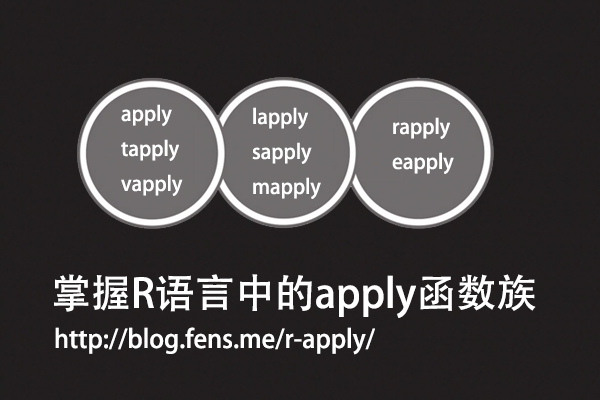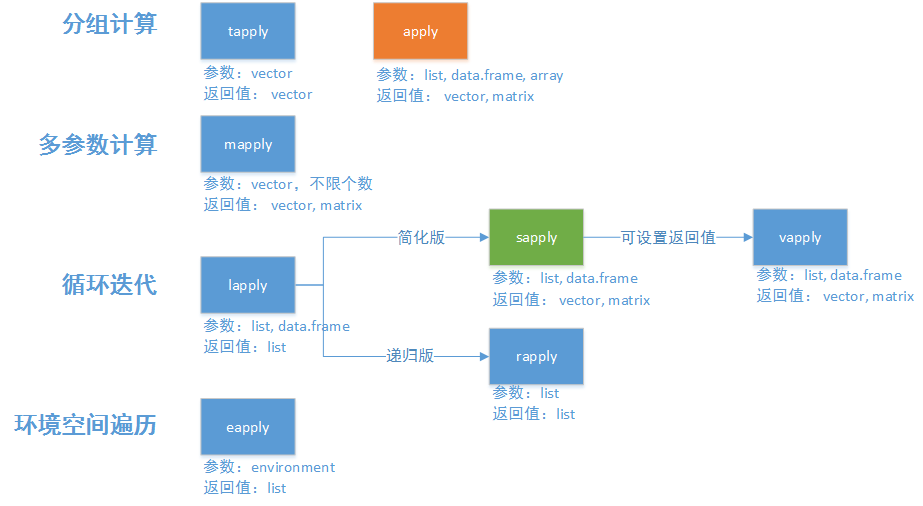• Posts tagged "for"

# Blog Archives

## 掌握R语言中的apply函数族

R的极客理想系列文章，涵盖了R的思想，使用，工具，创新等的一系列要点，以我个人的学习和体验去诠释R的强大。

R语言作为统计学一门语言，一直在小众领域闪耀着光芒。直到大数据的爆发，R语言变成了一门炙手可热的数据分析的利器。随着越来越多的工程背景的人的加入，R语言的社区在迅速扩大成长。现在已不仅仅是统计领域，教育，银行，电商，互联网….都在使用R语言。

• 张丹(Conan), 程序员Java,R,PHP,Javascript
• weibo：@Conan_Z
• blog: http://blog.fens.me
• email: bsspirit@gmail.com

http://blog.fens.me/r-apply/1. apply的家族函数
2. apply函数
3. lapply函数
4. sapply函数
5. vapply函数
6. mapply函数
7. tapply函数
8. rapply函数
9. eapply函数

## 1. apply的家族函数

apply函数族是R语言中数据处理的一组核心函数，通过使用apply函数，我们可以实现对数据的循环、分组、过滤、类型控制等操作。但是，由于在R语言中apply函数与其他语言循环体的处理思路是完全不一样的，所以apply函数族一直是使用者玩不转一类核心函数。

apply函数本身就是解决数据循环处理的问题，为了面向不同的数据类型，不同的返回值，apply函数组成了一个函数族，包括了8个功能类似的函数。这其中有些函数很相似，有些也不是太一样的。## 2. apply函数

apply函数是最常用的代替for循环的函数。apply函数可以对矩阵、数据框、数组(二维、多维)，按行或列进行循环计算，对子元素进行迭代，并把子元素以参数传递的形式给自定义的FUN函数中，并以返回计算结果。

``apply(X, MARGIN, FUN, ...)``

• X:数组、矩阵、数据框
• MARGIN: 按行计算或按按列计算，1表示按行，2表示按列
• FUN: 自定义的调用函数
• …: 更多参数，可选

``````
> x<-matrix(1:12,ncol=3)
> apply(x,1,sum)
 15 18 21 24
``````

``````
# 生成data.frame
> x <- cbind(x1 = 3, x2 = c(4:1, 2:5)); x
x1 x2
[1,]  3  4
[2,]  3  3
[3,]  3  2
[4,]  3  1
[5,]  3  2
[6,]  3  3
[7,]  3  4
[8,]  3  5

# 自定义函数myFUN，第一个参数x为数据
# 第二、三个参数为自定义参数，可以通过apply的'...'进行传入。
> myFUN<- function(x, c1, c2) {
+   c(sum(x[c1],1), mean(x[c2]))
+ }

# 把数据框按行做循环，每行分别传递给myFUN函数，设置c1,c2对应myFUN的第二、三个参数
> apply(x,1,myFUN,c1='x1',c2=c('x1','x2'))
[,1] [,2] [,3] [,4] [,5] [,6] [,7] [,8]
[1,]  4.0    4  4.0    4  4.0    4  4.0    4
[2,]  3.5    3  2.5    2  2.5    3  3.5    4
``````

``````
# 定义一个结果的数据框
> df<-data.frame()

# 定义for循环
> for(i in 1:nrow(x)){
+   row<-x[i,]                                         # 每行的值
+   df<-rbind(df,rbind(c(sum(row,1), mean(row))))   # 计算，并赋值到结果数据框
+ }

# 打印结果数据框
> df
V1  V2
1  4 3.5
2  4 3.0
3  4 2.5
4  4 2.0
5  4 2.5
6  4 3.0
7  4 3.5
8  4 4.0
``````

``````
> data.frame(x1=x[,1]+1,x2=rowMeans(x))
x1  x2
1  4 3.5
2  4 3.0
3  4 2.5
4  4 2.0
5  4 2.5
6  4 3.0
7  4 3.5
8  4 4.0
``````

``````
# 清空环境变量
> rm(list=ls())

# 封装fun1
> fun1<-function(x){
+   myFUN<- function(x, c1, c2) {
+     c(sum(x[c1],1), mean(x[c2]))
+   }
+   apply(x,1,myFUN,c1='x1',c2=c('x1','x2'))
+ }

# 封装fun2
> fun2<-function(x){
+   df<-data.frame()
+   for(i in 1:nrow(x)){
+     row<-x[i,]
+     df<-rbind(df,rbind(c(sum(row,1), mean(row))))
+   }
+ }

# 封装fun3
> fun3<-function(x){
+   data.frame(x1=x[,1]+1,x2=rowMeans(x))
+ }

# 生成数据集
> x <- cbind(x1=3, x2 = c(400:1, 2:500))

# 分别统计3种方法的CPU耗时。
> system.time(fun1(x))

0.01 0.00 0.02

> system.time(fun2(x))

0.19 0.00 0.18

> system.time(fun3(x))

0    0    0
``````

## 3. lapply函数

lapply函数是一个最基础循环操作函数之一，用来对list、data.frame数据集进行循环，并返回和X长度同样的list结构作为结果集，通过lapply的开头的第一个字母’l’就可以判断返回结果集的类型。

``lapply(X, FUN, ...)``

• X:list、data.frame数据
• FUN: 自定义的调用函数
• …: 更多参数，可选

``````
# 构建一个list数据集x，分别包括a,b,c 三个KEY值。
> x <- list(a = 1:10, b = rnorm(6,10,5), c = c(TRUE,FALSE,FALSE,TRUE));x
\$a
  1  2  3  4  5  6  7  8  9 10
\$b
  0.7585424 14.3662366 13.3772979 11.6658990  9.7011387 21.5321427
\$c
  TRUE FALSE FALSE  TRUE

# 分别计算每个KEY对应该的数据的分位数。
> lapply(x,fivenum)
\$a
  1.0  3.0  5.5  8.0 10.0

\$b
  0.7585424  9.7011387 12.5215985 14.3662366 21.5321427

\$c
 0.0 0.0 0.5 1.0 1.0
``````

lapply就可以很方便地把list数据集进行循环操作了，还可以用data.frame数据集按列进行循环，但如果传入的数据集是一个向量或矩阵对象，那么直接使用lapply就不能达到想要的效果了。

``````
# 生成一个矩阵
> x <- cbind(x1=3, x2=c(2:1,4:5))
> x; class(x)
x1 x2
[1,]  3  2
[2,]  3  1
[3,]  3  4
[4,]  3  5
 "matrix"

# 求和
> lapply(x, sum)
[]
 3

[]
 3

[]
 3

[]
 3

[]
 2

[]
 1

[]
 4

[]
 5
``````

lapply会分别循环矩阵中的每个值，而不是按行或按列进行分组计算。

``````
> lapply(data.frame(x), sum)
\$x1
 12

\$x2
 12
``````

lapply会自动把数据框按列进行分组，再进行计算。

## 4. sapply函数

sapply函数是一个简化版的lapply，sapply增加了2个参数simplify和USE.NAMES，主要就是让输出看起来更友好，返回值为向量，而不是list对象。

``sapply(X, FUN, ..., simplify=TRUE, USE.NAMES = TRUE)``

• X:数组、矩阵、数据框
• FUN: 自定义的调用函数
• …: 更多参数，可选
• simplify: 是否数组化，当值array时，输出结果按数组进行分组
• USE.NAMES: 如果X为字符串，TRUE设置字符串为数据名，FALSE不设置

``````
> x <- cbind(x1=3, x2=c(2:1,4:5))

# 对矩阵计算，计算过程同lapply函数
> sapply(x, sum)
 3 3 3 3 2 1 4 5

# 对数据框计算
> sapply(data.frame(x), sum)
x1 x2
12 12

# 检查结果类型，sapply返回类型为向量，而lapply的返回类型为list
> class(lapply(x, sum))
 "list"
> class(sapply(x, sum))
 "numeric"
``````

``````
> lapply(data.frame(x), sum)
\$x1
 12

\$x2
 12

> sapply(data.frame(x), sum, simplify=FALSE, USE.NAMES=FALSE)
\$x1
 12

\$x2
 12
``````

``````
> a<-1:2

# 按数组分组
> sapply(a,function(x) matrix(x,2,2), simplify='array')
, , 1

[,1] [,2]
[1,]    1    1
[2,]    1    1

, , 2

[,1] [,2]
[1,]    2    2
[2,]    2    2

# 默认情况，则自动合并分组
> sapply(a,function(x) matrix(x,2,2))
[,1] [,2]
[1,]    1    2
[2,]    1    2
[3,]    1    2
[4,]    1    2
``````

``````
> val<-head(letters)

# 默认设置数据名
> sapply(val,paste,USE.NAMES=TRUE)
a   b   c   d   e   f
"a" "b" "c" "d" "e" "f"

# USE.NAMES=FALSE，则不设置数据名
> sapply(val,paste,USE.NAMES=FALSE)
 "a" "b" "c" "d" "e" "f"
``````

## 5. vapply函数

vapply类似于sapply，提供了FUN.VALUE参数，用来控制返回值的行名，这样可以让程序更健壮。

``vapply(X, FUN, FUN.VALUE, ..., USE.NAMES = TRUE)``

• X:数组、矩阵、数据框
• FUN: 自定义的调用函数
• FUN.VALUE: 定义返回值的行名row.names
• …: 更多参数，可选
• USE.NAMES: 如果X为字符串，TRUE设置字符串为数据名，FALSE不设置

``````
# 生成数据集
> x <- data.frame(cbind(x1=3, x2=c(2:1,4:5)))

# 设置行名，4行分别为a,b,c,d
> vapply(x,cumsum,FUN.VALUE=c('a'=0,'b'=0,'c'=0,'d'=0))
x1 x2
a  3  2
b  6  3
c  9  7
d 12 12

# 当不设置时，为默认的索引值
> a<-sapply(x,cumsum);a
x1 x2
[1,]  3  2
[2,]  6  3
[3,]  9  7
[4,] 12 12

# 手动的方式设置行名
> row.names(a)<-c('a','b','c','d')
> a
x1 x2
a  3  2
b  6  3
c  9  7
d 12 12
``````

## 6. mapply函数

mapply也是sapply的变形函数，类似多变量的sapply，但是参数定义有些变化。第一参数为自定义的FUN函数，第二个参数’…’可以接收多个数据，作为FUN函数的参数调用。

``mapply(FUN, ..., MoreArgs = NULL, SIMPLIFY = TRUE,USE.NAMES = TRUE)``

• FUN: 自定义的调用函数
• …: 接收多个数据
• MoreArgs: 参数列表
• SIMPLIFY: 是否数组化，当值array时，输出结果按数组进行分组
• USE.NAMES: 如果X为字符串，TRUE设置字符串为数据名，FALSE不设置

``````
> set.seed(1)

# 定义3个向量
> x<-1:10
> y<-5:-4
> z<-round(runif(10,-5,5))

# 按索引顺序取较大的值。
> mapply(max,x,y,z)
  5  4  3  4  5  6  7  8  9 10
``````

``````
> set.seed(1)

# 长度为4
> n<-rep(4,4)

# m为均值，v为方差
> m<-v<-c(1,10,100,1000)

# 生成4组数据，按列分组
> mapply(rnorm,n,m,v)
[,1]      [,2]      [,3]       [,4]
[1,] 0.3735462 13.295078 157.57814   378.7594
[2,] 1.1836433  1.795316  69.46116 -1214.6999
[3,] 0.1643714 14.874291 251.17812  2124.9309
[4,] 2.5952808 17.383247 138.98432   955.0664
``````

## 7. tapply函数

tapply用于分组的循环计算，通过INDEX参数可以把数据集X进行分组，相当于group by的操作。

``tapply(X, INDEX, FUN = NULL, ..., simplify = TRUE)``

• X: 向量
• INDEX: 用于分组的索引
• FUN: 自定义的调用函数
• …: 接收多个数据
• simplify : 是否数组化，当值array时，输出结果按数组进行分组

``````
# 通过iris\$Species品种进行分组
> tapply(iris\$Petal.Length,iris\$Species,mean)
setosa versicolor  virginica
1.462      4.260      5.552
``````

``````
> set.seed(1)

# 定义x,y向量
> x<-y<-1:10;x;y
  1  2  3  4  5  6  7  8  9 10
  1  2  3  4  5  6  7  8  9 10

# 设置分组索引t
> t<-round(runif(10,1,100)%%2);t
 1 2 2 1 1 2 1 0 1 1

# 对x进行分组求和
> tapply(x,t,sum)
0  1  2
8 36 11
``````

``````
> tapply(x,t,sum,y)
0  1  2
63 91 66
``````

## 8. rapply函数

rapply是一个递归版本的lapply，它只处理list类型数据，对list的每个元素进行递归遍历，如果list包括子元素则继续遍历。

``rapply(object, f, classes = "ANY", deflt = NULL, how = c("unlist", "replace", "list"), ...)``

• object:list数据
• f: 自定义的调用函数
• classes : 匹配类型, ANY为所有类型
• deflt: 非匹配类型的默认值
• how: 3种操作方式，当为replace时，则用调用f后的结果替换原list中原来的元素；当为list时，新建一个list，类型匹配调用f函数，不匹配赋值为deflt；当为unlist时，会执行一次unlist(recursive = TRUE)的操作
• …: 更多参数，可选

``````
> x=list(a=12,b=1:4,c=c('b','a'))
> y=pi
> z=data.frame(a=rnorm(10),b=1:10)
> a <- list(x=x,y=y,z=z)

# 进行排序，并替换原list的值
> rapply(a,sort, classes='numeric',how='replace')
\$x
\$x\$a
 12
\$x\$b
 4 3 2 1
\$x\$c
 "b" "a"

\$y
 3.141593

\$z
\$z\$a
 -0.8356286 -0.8204684 -0.6264538 -0.3053884  0.1836433  0.3295078
  0.4874291  0.5757814  0.7383247  1.5952808
\$z\$b
 10  9  8  7  6  5  4  3  2  1

> class(a\$z\$b)
 "integer"
``````

``````
> rapply(a,function(x) paste(x,'++++'),classes="character",deflt=NA, how = "list")
\$x
\$x\$a
 NA
\$x\$b
 NA
\$x\$c
 "b ++++" "a ++++"

\$y
 NA

\$z
\$z\$a
 NA
\$z\$b
 NA
``````

## 9. eapply函数

``eapply(env, FUN, ..., all.names = FALSE, USE.NAMES = TRUE)``

• env: 环境空间
• FUN: 自定义的调用函数
• …: 更多参数，可选
• all.names: 匹配类型, ANY为所有类型
• USE.NAMES: 如果X为字符串，TRUE设置字符串为数据名，FALSE不设置

``````
# 定义一个环境空间
> env

# 向这个环境空间中存入3个变量
> env\$a <- 1:10
> env\$beta <- exp(-3:3)
> env\$logic <- c(TRUE, FALSE, FALSE, TRUE)
> env

# 查看env空间中的变量
> ls(env)
 "a"     "beta"  "logic"

# 查看env空间中的变量字符串结构
> ls.str(env)
a :  int [1:10] 1 2 3 4 5 6 7 8 9 10
beta :  num [1:7] 0.0498 0.1353 0.3679 1 2.7183 ...
logic :  logi [1:4] TRUE FALSE FALSE TRUE
``````

``````
> eapply(env, mean)
\$logic
 0.5
\$beta
 4.535125
\$a
 5.5
``````

``````
# 查看当前环境空间中的变量
> ls()
 "a"     "df"     "env"    "x"     "y"    "z"    "X"

# 查看所有变量的占用内存大小
> eapply(environment(), object.size)
\$a
2056 bytes

\$df
1576 bytes

\$x
656 bytes

\$y
48 bytes

\$z
952 bytes

\$X
1088 bytes

\$env
56 bytes
``````

eapply函数平时很难被用到，但对于R包开发来说，环境空间的使用是必须要掌握的。特别是当R要做为工业化的工具时，对变量的精确控制和管理是非常必要的。

http://blog.fens.me/r-apply/